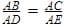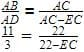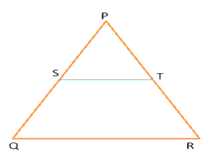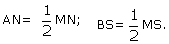Test: Converse of BPT

# Test: Converse of BPT - Class 10

Test Description

## 10 Questions MCQ Test Mathematics (Maths) Class 10 - Test: Converse of BPT

Test: Converse of BPT for Class 10 2023 is part of Mathematics (Maths) Class 10 preparation. The Test: Converse of BPT questions and answers have been prepared according to the Class 10 exam syllabus.The Test: Converse of BPT MCQs are made for Class 10 2023 Exam. Find important definitions, questions, notes, meanings, examples, exercises, MCQs and online tests for Test: Converse of BPT below.
Solutions of Test: Converse of BPT questions in English are available as part of our Mathematics (Maths) Class 10 for Class 10 & Test: Converse of BPT solutions in Hindi for Mathematics (Maths) Class 10 course. Download more important topics, notes, lectures and mock test series for Class 10 Exam by signing up for free. Attempt Test: Converse of BPT | 10 questions in 10 minutes | Mock test for Class 10 preparation | Free important questions MCQ to study Mathematics (Maths) Class 10 for Class 10 Exam | Download free PDF with solutions
 1 Crore+ students have signed up on EduRev. Have you?
Test: Converse of BPT - Question 1

### In triangle ABC ,DE || BC AD=3 cm, DB = 8 cm AC = 22 cm. At what distance from A does the line DE cut AC?

Detailed Solution for Test: Converse of BPT - Question 1

In triangle ABC and ADE, Angle A is common and since DE || BC, Angle D= Angle B. So by  AA criterion, ΔABC - ΔADE
So,EC=16
AE=AC-EC=6cm

Test: Converse of BPT - Question 2

### In a triangle ABC, BC || DE If AD = 1.5, DB = 4.5, AE = 1.3, EC = ?​

Test: Converse of BPT - Question 3

### In triangle DEF,GH is a line parallel to EF cutting DE in G and and DF in H. If DE = 16.5, DH = 5, HF = 6 GE = ?

Test: Converse of BPT - Question 4

In triangle ABC, DE || BC, AD = 1.2 cm DB = 3.6 cm, AE = 1.6 cm.What must be the value of EC?​

Test: Converse of BPT - Question 5

In trapezium ABCD a line EF cuts the diagonal AC in O such that AO/OC = 2/3 and EF is parallel to BC.In what ratio does EF cut AB and CD?​

Test: Converse of BPT - Question 6

In the given figure S and T are respectively points on the side PQ and PR of a ΔPQR. If PS = 2.5 cm, PQ = 7.5 cm, PT = 1.7 cm and PR = 5.1 cm then find that which of the following relations definitely holds trueTest: Converse of BPT - Question 7

In triangle MNS, A and B are points on the sides MN, NS respectively.Then AB is …………………. to NS :​

Detailed Solution for Test: Converse of BPT - Question 7

In ∆MNS we have,

→ AN = 1/2(MN)
→ AN = (1/2)(MA + AN)
→ AN = (1/2)MA + (1/2)AN
→ AN - (1/2)AN = (1/2)MA
→ AN[1 - (1/2)] = (1/2)MA
→ (1/2)AN = (1/2)MA
→ AN = MA
so, A is mid point of MN .

similarly,
→ BS = (1/2)(MS)

so, B is mid point of MS .

since AB is a line segment joining the mid points of two sides of ∆MNS .

therefore, → AB || NS { According to Mid point theorem the line segment joining the mid points of two sides of a triangle is parallel to the third side . }

Test: Converse of BPT - Question 8

Triangle ABC is an isosceles right triangle right angled at B.DE is a line parallel to BC which intersects AB in D and AC in E.What ratio are the sides of triangle ADE in?

Detailed Solution for Test: Converse of BPT - Question 8

Since AB =BC and the triangles are similar, AB/AD=BC/DE
So by Pythagoras theorem,
H2 = P2 + B2
H= 2x2
H = √2x
So the sides are in the ratio x:x: √2x which is 1:1: √2

Test: Converse of BPT - Question 9

Two poles stand on the ground at a distance of 20m and 50 m respectively from a point A on the ground, the taller pole at 30 m from smaller pole. A cable originates from the top pf the taller pole, passing on the other pole ends on a hook at point A. If the length of the cable is 100 m , how much of it lies between the the two poles?

Test: Converse of BPT - Question 10

In triangle MNP a line QR intersects MN and MP in Q and R respectivelysuch that MQ/QN = MR/RP. If ∠MNP = 60°, ∠MPN = 50° What are the values of the angles of the triangle MQR?​

## Mathematics (Maths) Class 10

115 videos|478 docs|129 tests
Information about Test: Converse of BPT Page
In this test you can find the Exam questions for Test: Converse of BPT solved & explained in the simplest way possible. Besides giving Questions and answers for Test: Converse of BPT, EduRev gives you an ample number of Online tests for practice

## Mathematics (Maths) Class 10

115 videos|478 docs|129 tests